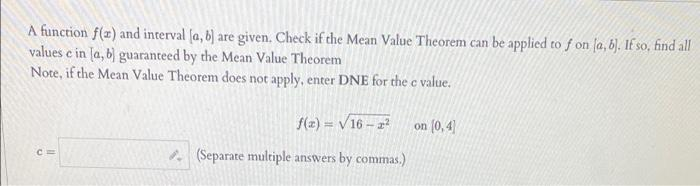# Question Solved1 Answer A function f(x) and interval [a, b] are given. Check if the Mean Value Theorem can be applied to f on [a, b]. If so, find all values e in [a, b] guaranteed by the Mean Value Theorem Note, if the Mean Value Theorem does not apply, enter DNE for the c value. f(x)=√16-2² on (0,4) CO (Separate multiple answers by commas.) A function f(x) and interval [a, b] are given. Check if the Mean Value Theorem can be applied to f on [a, b]. If so, find all values e in [a, b] guaranteed by the Mean Value Theorem Note, if the Mean Value Theorem does not apply, enter DNE for the c value. f(x)=√16-2² on (0,4) CO (Separate multiple answers by commas.)Transcribed Image Text: A function f(x) and interval [a, b] are given. Check if the Mean Value Theorem can be applied to f on [a, b]. If so, find all values e in [a, b] guaranteed by the Mean Value Theorem Note, if the Mean Value Theorem does not apply, enter DNE for the c value. f(x)=√16-2² on (0,4) CO (Separate multiple answers by commas.)
More
Transcribed Image Text: A function f(x) and interval [a, b] are given. Check if the Mean Value Theorem can be applied to f on [a, b]. If so, find all values e in [a, b] guaranteed by the Mean Value Theorem Note, if the Mean Value Theorem does not apply, enter DNE for the c value. f(x)=√16-2² on (0,4) CO (Separate multiple answers by commas.)